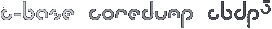#### Site Tools

projects:c-base-sign

This is an old revision of the document!

``` /* control for the LEDs in the nerdctrl c-base sign Works with TI's connected launchpad and the energia.nu IDE. */ 80 C red 37 C blue 78 B red 10 B green 79 B blue 40 A red 38 A green 9 A blue 55 S red 77 S green 19 S blue 54 E red 76 E green 75 E blue #define C_RED 80 #define C_BLUE 37 #define B_RED 78 #define B_BLUE 79 #define A_RED 40 #define A_BLUE 9 #define S_RED 55 #define S_BLUE 19 #define E_RED 54 #define E_BLUE 75 int brightness = 50; how bright the LED is int fadeAmount = 1; how many points to fade the LED by int mode = 1; int red[] = {80, 78, 40, 55, 54}; int green[] = {42, 10, 38, 77, 76}; int blue[] = {37, 79, 9, 19, 75}; void setup() { Serial.begin(9600); for (int i=0; i<5; i++) { pinMode(red[i], OUTPUT); pinMode(green[i], OUTPUT); pinMode(blue[i], OUTPUT); } } void loop() { while (Serial.available() > 0) { mode = Serial.parseInt(); brightness = 255; Serial.println(“aye”); } if (brightness < 90) { fadeAmount = 1; } if (brightness >= 255) { fadeAmount = -1; } if (mode == 0) { for (int i=0; i<5; i++) { analogWrite(red[i], 0); analogWrite(green[i], 0); analogWrite(blue[i], 0); } } else if (mode == 1) { for (int i=0; i<5; i++) { analogWrite(red[i], 0); analogWrite(green[i], 0); analogWrite(blue[i], brightness); } } else if (mode == 2) { for (int i=0; i<5; i++) { analogWrite(red[i], brightness); analogWrite(green[i], 0); analogWrite(blue[i], 0); } } else if (mode == 3) { for (int i=0; i<5; i++) { analogWrite(red[i], 0); analogWrite(green[i], brightness); analogWrite(blue[i], 0); } } else if (mode == 4) { for (int i=0; i<5; i++) { analogWrite(red[i], brightness); analogWrite(green[i], 0); analogWrite(blue[i], brightness); } } else if (mode == 5) { for (int i=0; i<5; i++) { analogWrite(red[i], 0); analogWrite(green[i], brightness); analogWrite(blue[i], brightness); } } else if (mode == 6) { for (int i=0; i<5; i++) { analogWrite(red[i], brightness); analogWrite(green[i], brightness); analogWrite(blue[i], 0); } } else if (mode == 7) { for (int i=0; i<5; i++) { analogWrite(red[i], brightness); analogWrite(green[i], brightness); analogWrite(blue[i], brightness); } } delay(10); brightness = brightness + fadeAmount; } ```